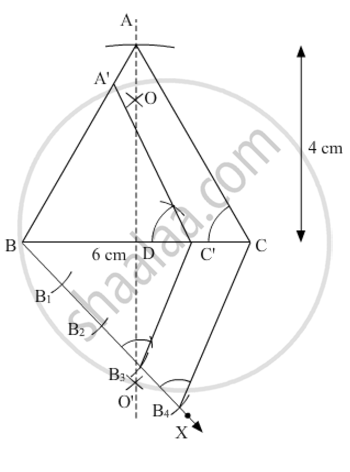# Construct an isosceles triangle whose base is 6 cm and altitude 4 cm. Then construct another triangle whose sides are 3/4times the corresponding sides of the isosceles triangle. - Mathematics

Construct an isosceles triangle whose base is 6 cm and altitude 4 cm. Then construct another triangle whose sides are 3/4times the corresponding sides of the isosceles triangle.

#### Solution

Let ABC be an isosceles triangle with AB = AC, base BC = 6 cm and altitude AD = 4 cm.

A ΔA'BC', whose sides are 34 times the sides of ΔABC, can be drawn using the following steps:

1. Draw a line segment BC of 6 cm. Draw arcs of the same radius on both sides of the line segment while taking points B and C as its centre. Let these arcs intersect each other at O and O'. Join OO'. Let OO' intersect BC at D

2. Taking D as centre, draw an arc of 4 cm radius which cuts the line segment OO' at point A. An isosceles ΔABC is formed, having altitude (AD) as 4 cm and base (BC) as 6 cm.

3. Draw a ray BX making an acute angle with line segment BC on the opposite side of vertex A.

4. Locate 4 points (as 4 is greater between 3 and 4) B1, B2, B3 and B4 on BX such that BB1 = B1B2 = B2B3 = B3B4.

5. Join CB4 and draw a line through B3 parallel to CB4 to intersect line segment BC at point C'.

6. Draw a line through C' parallel to AC intersecting line segment AB at A'.

Thus, ΔA'BC' is the required triangleConcept: Constructions Examples and Solutions
Is there an error in this question or solution?